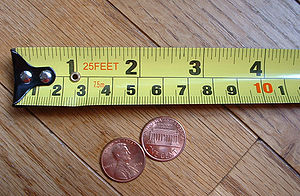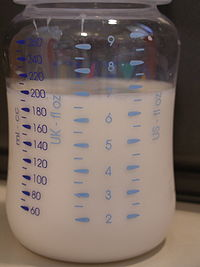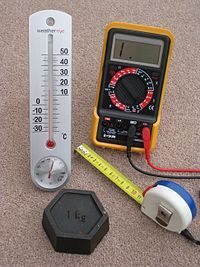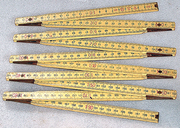# Measurement

﻿
MeasurementA typical tape measure with both metric and US units and two US pennies for comparison

Measurement is the process or the result of determining the ratio of a physical quantity, such as a length, time, temperature etc., to a unit of measurement, such as the metre, second or degree Celsius. The science of measurement is called metrology.

The English word measurement originates from the Latin mēnsūra and the verb metiri through the Middle French mesure.

## StandardsDenmark's national copy of the International Prototype Kilogram, delivered to Denmark in 1949 with an official mass of 1 kg + 81 µg.

With the exception of a few seemingly fundamental quantum constants, units of measurement are essentially arbitrary; in other words, people make them up and then agree to use them. Nothing inherent in nature dictates that an inch has to be a certain length, or that a mile is a better measure of distance than a kilometre. Over the course of human history, however, first for convenience and then for necessity, standards of measurement evolved so that communities would have certain common benchmarks. Laws regulating measurement were originally developed to prevent fraud in commerce.

Today, units of measurement are generally defined on a scientific basis, overseen by governmental or supra-governmental agencies, and established in international treaties, pre-eminent of which is the General Conference on Weights and Measures (CGPM), established in 1875 by the Treaty of the metre and which oversees the International System of Units (SI) and which has custody of the International Prototype Kilogram. The metre, for example, was redefined in 1983 by the CGPM as the distance traveled by light in free space in 1⁄299,792,458 of a second while in 1960 the international yard was defined by the governments of the United States, United Kingdom, Australia and South Africa as being exactly 0.9144 metres.

In the United States, the National Institute of Standards and Technology (NIST), a division of the United States Department of Commerce, regulates commercial measurements. In the United Kingdom, the role is performed by the National Physical Laboratory (NPL), in Australia by the Commonwealth Scientific and Industrial Research Organisation, in South Africa by the Council for Scientific and Industrial Research and in India the National Physical Laboratory of India.

## Units and systemsA baby bottle that measures in all three measurement systems, Imperial (U.K.), U.S. customary, and metric.Four measuring devices having metric calibrations

### Imperial system

Before SI units were widely adopted around the world, the British systems of English units and later imperial units were used in Britain, the Commonwealth and the United States. The system came to be known as U.S. customary units in the United States and is still in use there and in a few Caribbean countries. These various systems of measurement have at times been called foot-pound-second systems after the Imperial units for length, weight and time even though the tons, hundredweights, gallons, and nautical miles, for example, are different for the U.S. units. Many Imperial units remain in use in Britain which has officially partially switched to the SI system. Road signs are still in miles, yards, miles per hour; milk, beer and cider are sold by the pint; people measure their height in feet and inches and their weight in stone and pounds, to give just a few examples. Imperial units are used in many other places, for example, in many Commonwealth countries that are considered metricated, land area is measured in acres and floor space in square feet, particularly for commercial transactions (rather than government statistics). Similarly, gasoline is sold by the gallon in many countries that are considered metricated.

### Metric system

The metric system is a decimal systems of measurement based on its units for length, the metre and for mass, the kilogram. It exists in several variations, with different choices of base units, though these do not affect its day-to-day use. Since the 1960s, the International System of Units (SI) is the internationally recognized metric system. Metric units of mass, length, and electricity are widely used around the world for both everyday and scientific purposes.

The metric system features a single base unit for many physical quantities. Other quantities are derived from the standard SI units. Multiples and fractions of the units are expressed as powers of ten of each unit. Unit conversions are always simple because they are in the ratio of ten, one hundred, one thousand, etc., so that convenient magnitudes for measurements are achieved by simply moving the decimal place: 1.234 metres is 1234 millimetres or 0.001234 kilometres. The use of fractions, such as 2/5 of a metre, is not prohibited, but uncommon. All lengths and distances, for example, are measured in metres, or thousandths of a metre (millimetres), or thousands of metres (kilometres). There is no profusion of different units with different conversion factors as in the Imperial system which uses, for example, inches, feet, yards, fathoms, rods.

### International System of Units

The International System of Units (abbreviated as SI from the French language name Système International d'Unités) is the modern revision of the metric system. It is the world's most widely used system of units, both in everyday commerce and in science. The SI was developed in 1960 from the metre-kilogram-second (MKS) system, rather than the centimetre-gram-second (CGS) system, which, in turn, had many variants. During its development the SI also introduced several newly named units that were previously not a part of the metric system. The original SI units for the six basic physical quantities were:

• metre (m)  :SI unit of length
• second (s)  :SI unit of time
• kilogram (kg)  :SI unit of mass
• ampere (A)  :SI unit of electric current
• degree kelvin (K) :SI unit of thermodynamic temperature
• candela (cd)  :SI unit of luminous intensity

The mole was subsequently added to this list and the degree Kelvin renamed the kelvin.

There are two types of SI units, base units and derived units. Base units are the simple measurements for time, length, mass, temperature, amount of substance, electric current and light intensity. Derived units are constructed from the base units, for example, the watt, i.e. the unit for power, is defined from the base units as m2·kg·s−3. Other physical properties may be measured in compound units, such as material density, measured in kg/m3.

#### Converting prefixes

The SI allows easy multiplication when switching among units having the same base but different prefixes. To convert from metres to centimetres it is only necessary to multiply the number of metres by 100, since there are 100 centimetres in a metre. Inversely, to switch from centimetres to metres one multiplies the number of centimetres by 0.01 or divide centimetres by 100.

### LengthA 2-metre carpenter's ruler

A ruler or rule is a tool used in, for example, geometry, technical drawing, engineering, and carpentry, to measure lengths or distances or to draw straight lines. Strictly speaking, the ruler is the instrument used to rule straight lines and the calibrated instrument used for determining length is called a measure, however common usage calls both instruments rulers and the special name straightedge is used for an unmarked rule. The use of the word measure, in the sense of a measuring instrument, only survives in the phrase tape measure, an instrument that can be used to measure but cannot be used to draw straight lines. As can be seen in the photographs on this page, a two-metre carpenter's rule can be folded down to a length of only 20 centimetres, to easily fit in a pocket, and a five-metre-long tape measure easily retracts to fit within a small housing.

## Some special names

We also use some special names for some multiples of some units.

• 100 kilograms = 1 quintal; 1000 kilogram = 1 metric tonne;
• 10 years = 1 decade; 100 years = 1 century; 1000 years = 1 millennium

The Australian building trades adopted the metric system in 1966 and the units used for measurement of length are metres (m) and millimetres (mm). Centimetres (cm) are avoided as they cause confusion when reading plans. For example, the length two and a half metres is usually recorded as 2500 mm or 2.5 m; it would be considered non-standard to record this length as 250 cm.

American surveyors use a decimal-based system of measurement devised by Edmund Gunter in 1620. The base unit is Gunter's chain of 66 feet (20 m) which is subdivided into 4 rods, each of 16.5 ft or 100 links of 0.66 feet. A link is abbreviated "lk," and links "lks" in old deeds and Land Surveys done for the government.

### Time

Time is an abstract measurement we have invented in order to keep track of elemental changes over a non spatial continuum. It is denoted by numbers and/or named periods such as hours, days, weeks, or months. It is an apparently irreversible series of occurrences within this non spatial continuum. It is also used to denote an interval between two relative points on this continuum.

### Mass

Mass refers to the intrinsic property of all material objects to resist changes in their momentum. Weight, on the other hand, refers to the downward force produced when a mass is in a gravitational field. In free fall, (no net gravitational forces) objects lack weight but retain their mass. The Imperial units of mass include the ounce, pound, and ton. The metric units gram and kilogram are units of mass.

One device for measuring weight or mass is called a weighing scale or, often, simply a scale. A spring scale measures force but not mass, a balance compares weight, both require a gravitational field to operate. Some of the most accurate instruments for measuring weight or mass are based on load cells with a digital read-out, but require a gravitational field to function and would not work in free fall.

### Economics

The measures used in economics are physical measures, nominal price value measures and fixed price value measures. These measures differ from one another by the variables they measure and by the variables excluded from measurements. The measurable variables in economics are quantity, quality and distribution. By excluding variables from measurement makes it possible to better focus the measurement on a given variable, yet, this means a narrower approach.

## Difficulties

Since accurate measurement is essential in many fields, and since all measurements are necessarily approximations, a great deal of effort must be taken to make measurements as accurate as possible. For example, consider the problem of measuring the time it takes an object to fall a distance of one metre (about 39 in). Using physics, it can be shown that, in the gravitational field of the Earth, it should take any object about 0.45 second to fall one metre. However, the following are just some of the sources of error that arise:

1. This computation used for the acceleration of gravity 9.8 metres per second squared (32 ft/s2). But this measurement is not exact, but only precise to two significant digits.
2. The Earth's gravitational field varies slightly depending on height above sea level and other factors.
3. The computation of .45 seconds involved extracting a square root, a mathematical operation that required rounding off to some number of significant digits, in this case two significant digits.

So far, we have only considered scientific sources of error. In actual practice, dropping an object from a height of a metre stick and using a stopwatch to time its fall, we have other sources of error:

1. Most common, is simple carelessness.
2. Determining the exact time at which the object is released and the exact time it hits the ground. There is also the problem that the measurement of the height and the measurement of the time both involve some error.
3. Air resistance

Scientific experiments must be carried out with great care to eliminate as much error as possible, and to keep error estimates realistic.

## Definitions and theories

### Classical definition

In the classical definition, which is standard throughout the physical sciences, measurement is the determination or estimation of ratios of quantities. Quantity and measurement are mutually defined: quantitative attributes are those possible to measure, at least in principle. The classical concept of quantity can be traced back to John Wallis and Isaac Newton, and was foreshadowed in Euclid's Elements.

### Representational theory

In the representational theory, measurement is defined as "the correlation of numbers with entities that are not numbers". The most technically elaborate form of representational theory is also known as additive conjoint measurement. In this form of representational theory, numbers are assigned based on correspondences or similarities between the structure of number systems and the structure of qualitative systems. A property is quantitative if such structural similarities can be established. In weaker forms of representational theory, such as that implicit within the work of Stanley Smith Stevens, numbers need only be assigned according to a rule.

The concept of measurement is often misunderstood as merely the assignment of a value, but it is possible to assign a value in a way that is not a measurement in terms of the requirements of additive conjoint measurement. One may assign a value to a person's height, but unless it can be established that there is a correlation between measurements of height and empirical relations, it is not a measurement according to additive conjoint measurement theory. Likewise, computing and assigning arbitrary values, like the "book value" of an asset in accounting, is not a measurement because it does not satisfy the necessary criteria.

### Information theory

Information theory recognizes that all data are inexact and statistical in nature. Thus the definition of measurement is: "A set of observations that reduce uncertainty where the result is expressed as a quantity." This definition is implied in what scientists actually do when they measure something and report both the mean and statistics of the measurements. In practical terms, one begins with an initial guess as to the value of a quantity, and then, using various methods and instruments, reduces the uncertainty in the value. Note that in this view, unlike the positivist representational theory, all measurements are uncertain, so instead of assigning one value, a range of values is assigned to a measurement. This also implies that there is not a clear or neat distinction between estimation and measurement. Ascertaining the degree measurement error is also a basic facet of metrology, and sources of errors are divided into systematic and non-systematic.

### Quantum mechanics

In quantum mechanics, a measurement is an action that determines the location of an object, its momentum, its polarity (if it is a photon), etc. Before a measurement is made, the wavefunction of what is to be measured gives a range of probabilities for the outcomes of measurement, but when a measurement is accomplished that results in what is called the collapse of the wavefunction− at which time there is one definite value rather than a range of possible values.[citation needed] The unambiguous meaning of the measurement problem is an unresolved fundamental problem in quantum mechanics.[citation needed]

Wikimedia Foundation. 2010.

Synonyms:

### Look at other dictionaries:

• Measurement — measurement …   Philosophy dictionary

• Measurement — measurement …   Dictionary of sociology

• measurement — raises four main issues for the sociologist: representation (how many properties of the empirical world can best be modelled?); uniqueness (how unique are the resulting measurement numbers?); appropriate statistics (what indices may legitimately… …   Dictionary of sociology

• measurement — UK US /ˈmeʒəmənt/ noun ► [C or U] the act of measuring something: »The measurement of performance, or effectiveness, is a constant concern in management research. accurate/precise/proper/detailed measurement »It is these specific characteristics… …   Financial and business terms

• Measurement — Meas ure*ment, n. 1. The act or result of measuring; mensuration; as, measurement is required. [1913 Webster] 2. The extent, size, capacity, amount. or quantity ascertained by measuring; as, its measurement is five acres. [1913 Webster] …   The Collaborative International Dictionary of English

• measurement — I noun admeasurement, amount, analysis, appraisal, appraisement, assessment, bulk, calculation, capaciousness, capacity, computation, dimensions, estimate, estimation, extent, gauge, girth, greatness, largeness, limit, magnitude, mass, measure,… …   Law dictionary

• measurement — [mezh′ərmənt] n. 1. a measuring or being measured; mensuration 2. extent, quality, or size as determined by measuring; dimension [a waist measurement of 32 inches] 3. a system of measuring or of measures …   English World dictionary

• measurement — 1751, act of measuring, from MEASURE (Cf. measure) (v.) + MENT (Cf. ment). Related: Measurements. Meaning dimension obtained by measuring is from 1756 …   Etymology dictionary

• measurement — [n] calculation altitude, amount, amplitude, analysis, appraisal, area, assessment, calibration, capacity, computation, degree, density, depth, determination, dimension, distance, estimation, evaluation, extent, frequency, height, judgment,… …   New thesaurus

• measurement — ► NOUN 1) the action of measuring. 2) an amount, size, or extent found by measuring. 3) a standard unit used in measuring …   English terms dictionary